# Octagon

In geometry, Octagon is a polygon that has 8 sides and 8 angles. That means the number of vertices and edges of an octagon is 8, respectively. In simple words, the octagon is an 8-sided polygon, also called 8-gon, in a two-dimensional plane. A regular octagon will have all its sides equal in length. Each interior angle of a regular octagon is equal to 135°. Therefore, the measure of exterior angle becomes 180° – 135° = 45°. The sum of the interior angles of the octagon is 135 × 8 = 1080°. In this article, let us discuss the octagon shape, its formulas, properties, and examples in detail.

## What is an Octagon?

An octagon is a closed two-dimensional figure with eight sides, eight vertices and eight interior angles. If all the sides and interior angles of an octagon are of equal measure, then it is called a regular octagon otherwise an irregular octagon. The other types of octagons such as convex and concave octagons are also explained in the next sections.

## Shape of Octagon

Octagon is a geometrical shape in a two-dimensional plane. Like the other polygon shapes, which we have studied in geometry, such as triangle, square, pentagon, hexagon, rectangle, etc., the octagon is also a polygon. The point which defines it as different from other geometrical shapes is that it has 8 sides and 8 angles.

If squares are built internally or externally on all the sides of an octagon, then the midpoints of the sections joining the centers of opposite squares form a quadrilateral: equi-diagonal and ortho-diagonal ( whose diagonals length are equal and they bisect each other at 90 degrees).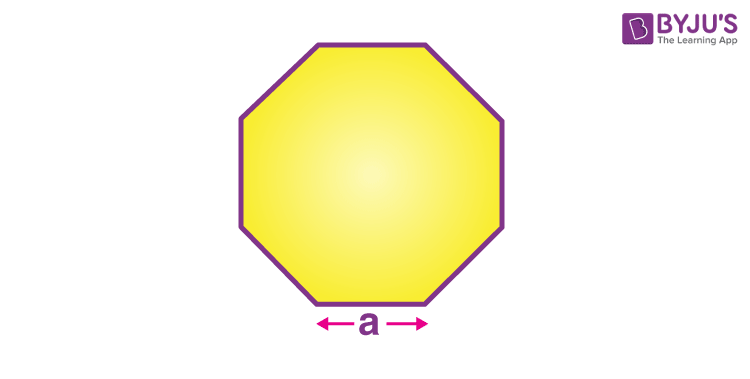You can see in the above figure, there are 8 sides of the polygon and eight vertices as well. This is a regular octagon because all the angles and sides here are equal.

### Real-life Examples of Octagon

We might have observed that different objects that we use in our everyday life contain an octagonal shape. Some of the examples include the following:

• Outline of an umbrella with 8 ribs
• Stop signboard at the signals
• A wall clock with 8 edges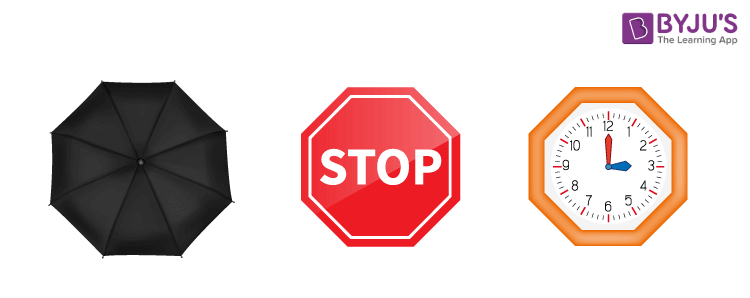## Octagon sides

In the previous section, you learned about the shape of an octagon with the help of an image. Let’s understand octagon sides and how to represent them.

How many sides does an octagon have?

As we already know, the octagon is an 8-sided polygon. That means an octagon contains 8 sides. Also, based on the length of these sides, octagons are classified as regular and irregular octagons.

## Octagon angles

As defined above, the octagonal shape contains 8 angles at 8 vertices. Thus, the octagon holds 8 sides and 8 angles. There are 8 interior angles and 8 exterior angles in an octagon. Octagon interior angles sum is equal to 1080 degrees. Also, the sum of all eight exterior angles is equal to 360 degrees. Based on the type of angles, octagons are classified as convex and concave octagons.

## Properties of Octagon

In the case of properties, we usually consider regular octagons.

• These have eight sides and eight angles.
• All the sides and all the angles are equal, respectively.
• There are a total of 20 diagonals in a regular octagon.
• The total sum of the interior angles is 1080°, where each angle is equal to 135°(135×8 = 1080)
• Sum of all the exterior angles of the octagon is 360°, and each angle is 45°(45×8=360).

## Types of Octagon

Depending upon the sides and angles of the octagon, it is classified into the following categories;

• Regular and Irregular Octagon
• Concave and Convex Octagon

### Regular and Irregular Octagon

When an octagon has all equal sides and equal angles, then it is defined as a regular octagon. But if it has unequal sides and unequal angles, it is defined as an irregular octagon. See the figure below to see the difference between them.

A regular octagon is a closed shape with sides of equal length and interior angles of the same measurement. It has eight symmetric lines and a rotational equilibrium of order 8. The interior angle at each vertex of a regular octagon is 135°. The central angle is 45°.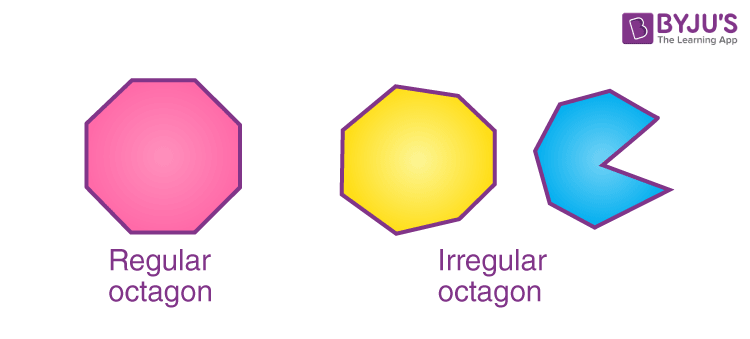In the above figure, the left-hand side figure depicts a regular octagon and the two figures on the right side shows irregular octagons. From the figure itself, we can analyse that there is a difference between the symmetry of regular and irregular polygons.

### Convex and Concave Octagon

The octagon which has all its angles pointing outside or no angles pointing inwards, is a convex octagon. The angles of the convex octagon are not more than 180°. And the octagon, with one of its angles pointing inward, is a concave-shaped octagon.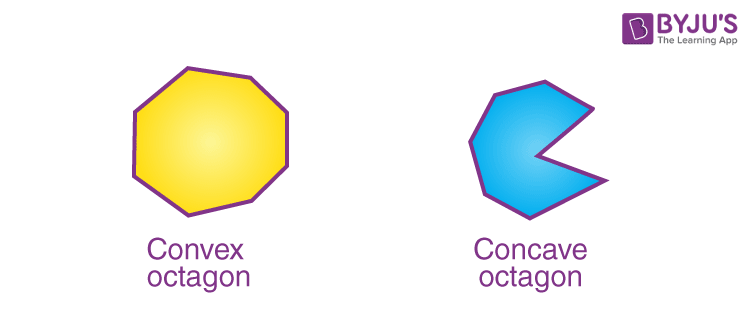In the above figure, you can see, the convex octagon has all its angles pointing outside from the center or origin point. Whereas on the right side, the concave octagon has one of the angles pointing

towards the inside of the polygon.

## Diagonals of Octagon

For any n-sided polygon, we can find the number of diagonals using the formula n(n – 3)/2.

Similarly, we can find the number of diagonals in an octagon.

For octagon, n = 8

Substituting n – 8 in the required formula, we get;

n(n – 3)/2 = 8(8 – 3)/2 = 4(5) = 20

Therefore, an octagon contains a total of 20 diagonals. These can be drawn as shown in the below figure.### Length of the Diagonal of Octagon

If we join the opposite vertices of a regular octagon, then the diagonals formed have the length equal to:

L = a√(4 + 2√2)

where a is the side of the octagon.

## Perimeter of Octagon

The perimeter of the octagon is the length of the sides or boundaries of the octagon, which forms a closed shape.

Therefore,

Perimeter = Sum of all Sides = 8a

Where a is the length of one side of the octagon.

## Area of Regular Octagon

The area of the octagon is the region covered by the sides of the octagon. The formula for the area of a regular octagon which has 8 equal sides and all its interior angles are equal to 135°, is given by:

Area = 2a2(1 + √2)
This is the octagon area formula in case of equal sides.

## Octagon Lines of symmetry

We know that the line of symmetry of a shape divides it into identical halves. Thus, being an 8 sided polygon, it has 8 lines of symmetry. However, these lines of symmetry can be drawn for regular octagons and this can be shown as: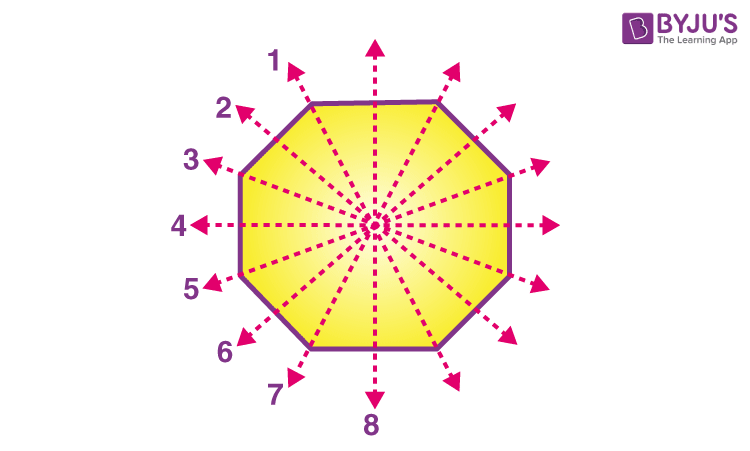## Octagonal Prism

An octagonal prism has two octagonal faces and eight square sides. The shape of an octagonal prism is given as: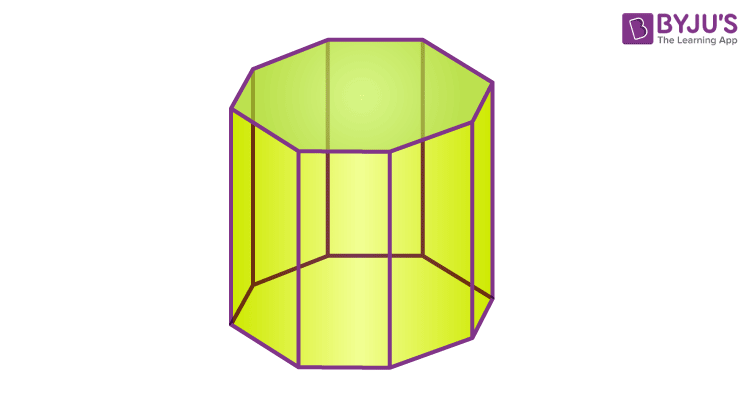## Octagonal Pyramid

If the base of a pyramid is an octagon, then we call it an octagonal pyramid. The shape of an octagonal pyramid is given in the figure below.## Solved Examples on Octagon

Q.1: If the length of the side of a regular octagon is 5 cm. Find its perimeter and area.

Solution:

Given, a = 5 cm

Therefore, Perimeter = 8a = 8 × 5 = 40 cm

And Area = 2a2(1+√2) = 2 × 52 (1+√2) = 2 × 25 (1+√2)= 120.7 cm2

Q.2: If the side length of a regular octagon is 7 cm. Find its area.

Solution:

Given, length of the side of the octagon, a = 7 cm

Area = 2a2(1+√2) = 2 (7)2(1+√2) =  236.6 sq.cm.

Q.3: Find the length of the longest diagonal of a regular octagon whose side length is equal to 10 cm.

Solution:

Given,

Length of side of octagon = a = 10 cm

By the formula, we know, the length of the longest diagonal formula is given by:

L = a√(4 + 2√2)

Hence,

L = 10√(4 + 2√2)

L = 10 x √6.828

L= 10 x 2.613

L = 26.13 cm

Q.4: Find the area and perimeter of a regular octagon whose side is of length 2.5 cm.

Solution:

Given,

Length of side of octagon = a = 2.5 cm

Area of octagon = 2a2(1+√2)

A = 2 x (2.5)(1+√2)

A = 12.5 x (1+√2)

A = 30.177 sq.cm

Perimeter of Octagon = 8 x sides of the octagon

P = 8 x 2.5

P = 20cm

## Frequently Asked Questions on Octagon

Q1

### What is the shape of the octagon?

In geometry, an octagon is a polygon that has 8 sides and 8 angles. All the sides are joined end to end to form the shape of the octagon. The sum of the interior angles of an octagon is equal to 1080 degrees.

Q2

### What are the different classifications of the octagon?

The octagon can be classified into:
Regular and irregular octagon
Convex and concave octagon

Q3

### What is meant by the regular and irregular octagon?

If all the sides and angles of an octagon are of the same measure, then it is called the regular octagon. If the octagon has unequal side length and angle, then it is called an irregular octagon.

Q4

### Define convex and concave octagon.

If all the angles of an octagon are pointing outwards and the interior angles are not more than 180 degrees, then it is called a convex polygon. If any of the angles are pointing inwards, then it is called a concave polygon.

Q5

### Mention the properties of the octagon.

The important properties of the octagon are:
Octagon has 8 sides and 8 angles
The sum of the interior angles of an octagon is equal to 1080 degrees.
There are 20 diagonals in a regular octagon.

Download BYJU’S-The Learning App and understand the different types of geometrical concepts with the help of pictures and videos.

Quiz on Octagon